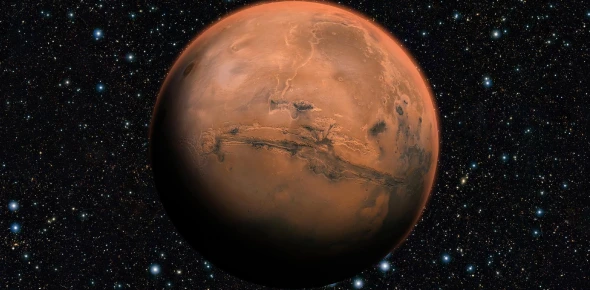Approved & Edited by ProProfs Editorial Team
At ProProfs Quizzes, our dedicated in-house team of experts takes pride in their work. With a sharp eye for detail, they meticulously review each quiz. This ensures that every quiz, taken by over 100 million users, meets our standards of accuracy, clarity, and engagement.
| Written by Aboyle
A
Aboyle
Community Contributor
Quizzes Created: 1 | Total Attempts: 5,419
Questions: 10 | Attempts: 5,419SettingsMars, named after the god of war in Roman mythology, is one of the five planets well known to many of the world's ancient civilizations. Throughout history, it's been the subject of observation and speculation. But how much do you know about Earth's neighbor? This quiz just might teach you a thing or two about the "fourth rock from the Sun."

• 1.

### How does Mars' diameter compare with Earth's?

• A.

• B.

• C.

• D.

Explanation
Mars' average diameter is 4,217 miles, about half that of Earth but twice the diameter of Earth's moon. Because of Mars' lighter density and the way diameter translates into volume, the planet's mass is a tenth of Earth's mass.

Rate this question:

• 2.

### About how long is a Martian day?

• A.

A little more than 12 hours

• B.

A little more than 24 hours

• C.

A little more than 48 hours

• D.

The Martian day never ends

B. A little more than 24 hours
Explanation
The Martian day is called a "sol," and lasts 24 hours, 37 minutes, 23 seconds.

Rate this question:

• 3.

### In Earth terms, about how long is a Martian year?

• A.

178 Earth days

• B.

365 Earth days

• C.

687 Earth days

• D.

942 Earth days

C. 687 Earth days
Explanation
The Martian year equals 687 Earth days. Mars' poles are tilted 25 degrees, creating seasons similar to Earth's but twice as long.

Rate this question:

• 4.

### What is the dominant ingredient of the Martian atmosphere?

• A.

Oxygen

• B.

Nitrogen

• C.

Methane

• D.

Carbon dioxide

D. Carbon dioxide
Explanation
Carbon dioxide makes up more than 95 percent of the Martian atmosphere. The other ingredients include nitrogen (2.7 percent), argon (1.6 percent) and just a trace of oxygen.

Rate this question:

• 5.

### How high is the highest point on Mars?

• A.

4 miles high

• B.

10 miles high

• C.

16 miles high

• D.

120 miles high

C. 16 miles high
Explanation
Olympus Mons is a 16-mile-high volcano that covers about the same surface area as the state of Arizona. Mars also has the largest and deepest known canyons in the solar system, measuring as much as 2,500 miles long and six miles in relief from the canyon floor to the surrounding plateaus.

Rate this question:

• 6.

### If you were using radio signals to chat with a friend on Mars when the two planets were at their minimum distance from each other, about how long would the minimum gaps in the conversation be?

• A.

One minute

• B.

Six minutes

• C.

One hour

• D.

Two hours

B. Six minutes
Explanation
The minimum distance between Earth and Mars is 34.9 million miles, or about three light-minutes. Thus, the minimum round-trip travel time at the speed of light is six minutes. (When the distance between the two planets is widest, that figure would amount to about 45 minutes.)

Rate this question:

• 7.

### How many moons does Mars have?

• A.

None

• B.

One

• C.

Two

• D.

12

C. Two
Explanation
The Martian moons Phobos (Greek for "fear") and Deimos (Greek for "terror") measure only a few miles wide and are named after the mythological sons of Mars, the god of war.

Rate this question:

• 8.

### What's the average surface temperature of Mars in Fahrenheit degrees?

• A.

199 degrees

• B.

64 degrees

• C.

64 degrees below zero

• D.

199 degrees below zero

C. 64 degrees below zero
Explanation
The surface temperature varies from 199 below zero F during polar night to 63 degrees F at the Martian equator during midday at the closest point in orbit to the sun.

Rate this question:

• 9.

### If you weighed 100 pounds on Earth, about how much would you weigh on Mars?

• A.

10 pounds

• B.

38 pounds

• C.

50 pounds

• D.

100 pounds

B. 38 pounds
Explanation
Gravity on the Martian surface would be 38 percent of Earth's gravity, due to factors such as density and radius.

Rate this question:

• 10.

### Mars' surface environment would be deadly to animal life from Earth. Why?

• A.

Atmosphere

• B.

• C.

Neither of the above

• D.

Both of the aboveBack to top